Main Content

# grabcut

Segment image into foreground and background using iterative graph-based segmentation

## Syntax

``BW = grabcut(A,L,ROI)``
``BW = grabcut(A,L,ROI,foremask,backmask)``
``BW = grabcut(A,L,ROI,foreind,backind)``
``BW = grabcut(___,Name,Value)``

## Description

example

````BW = grabcut(A,L,ROI)` segments the image `A` into foreground and background regions. The label matrix `L` specifies the subregions of the image. `ROI` is a logical mask designating the initial region of interest.```
````BW = grabcut(A,L,ROI,foremask,backmask)` segments the image `A`, where `foremask` and `backmask` are masks designating pixels in the image as foreground and background, respectively.```
````BW = grabcut(A,L,ROI,foreind,backind)` segments the image `A`, where `foreind` and `backind` specify the linear indices of the pixels in the image marked as foreground and background, respectively.```
````BW = grabcut(___,Name,Value)` segments the image using name-value pairs to control aspects of the segmentation.```

## Examples

collapse all

Read an RGB image into the workspace.

`RGB = imread('peppers.png');`

Generate label matrix.

`L = superpixels(RGB,500);`

Specify a region of interest and create a mask image.

```figure imshow(RGB) h1 = drawpolygon(gca,'Position',[72,105; 1,231; 0,366; 104,359;... 394,307; 518,343; 510,39; 149,72]);``````roiPoints = h1.Position; roi = poly2mask(roiPoints(:,1),roiPoints(:,2),size(L,1),size(L,2));```

Perform the grab cut operation, specifying the original image, the label matrix and the ROI.

```BW = grabcut(RGB,L,roi); figure imshow(BW)```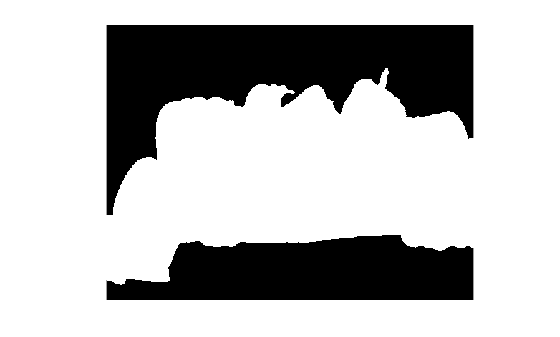Create masked image.

```maskedImage = RGB; maskedImage(repmat(~BW,[1 1 3])) = 0; figure; imshow(maskedImage)```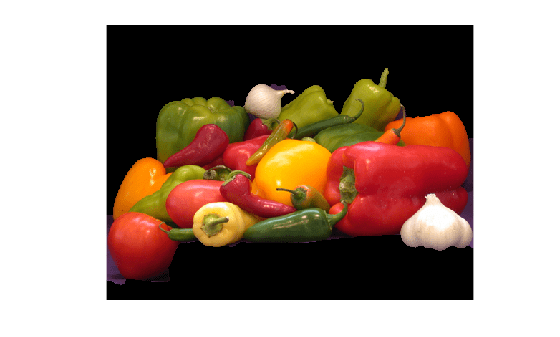Load 3-D volumetric data.

```load mristack V = mristack;```

Create a 2-D mask for initial foreground and background seed points.

```seedLevel = 10; fseed = V(:,:,seedLevel) > 75; bseed = V(:,:,seedLevel) == 0;```

Display foreground and background seed points.

`imshow(fseed)`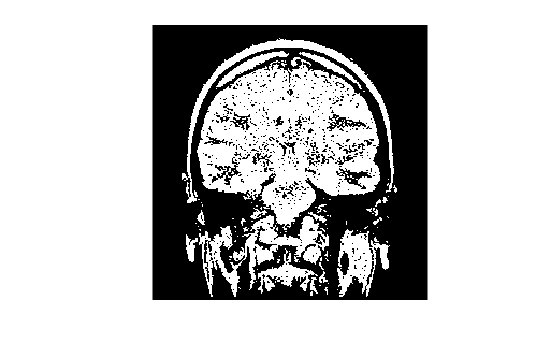`imshow(bseed)`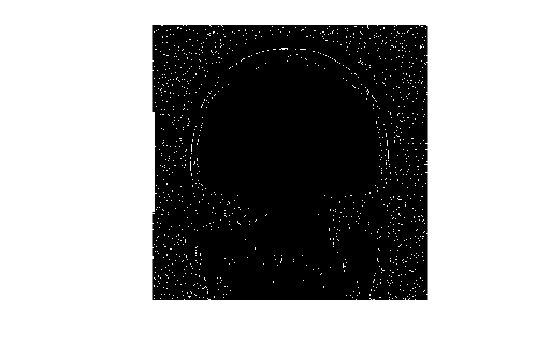Place seed points into empty 3-D mask.

```fmask = zeros(size(V)); bmask = fmask; fmask(:,:,seedLevel) = fseed; bmask(:,:,seedLevel) = bseed;```

Create initial region of interest.

```roi = false(size(V)); roi(10:end-10,10:end-10,:) = true;```

Generate label matrix.

`L = superpixels3(V,500);`

Perform GrabCut.

`bw = grabcut(V,L,roi,fmask,bmask);`

Display 3D segmented image.

`montage(reshape(bw,size(V)))`## Input Arguments

collapse all

Input image or volume, specified as a 2-D grayscale image, 2-D truecolor image, or 3-D grayscale volume. Only grayscale images can be data type `int16`.

Data Types: `single` | `double` | `int16` | `uint8` | `uint16`

Label matrix, specified as a numeric array.

Data Types: `single` | `double` | `int8` | `int16` | `int32` | `int64` | `uint8` | `uint16` | `uint32` | `uint64` | `logical`

Region of interest, specified as a logical array. All pixels that define the region of interest are equal to `true`.

Data Types: `logical`

Foreground mask, specified as a logical array.

Data Types: `single` | `double` | `int8` | `int16` | `int32` | `int64` | `uint8` | `uint16` | `uint32` | `uint64` | `logical`

Background mask, specified as a logical array.

Data Types: `single` | `double` | `int8` | `int16` | `int32` | `int64` | `uint8` | `uint16` | `uint32` | `uint64` | `logical`

Indices of pixels in foreground, specified as a vector of linear indices.

Data Types: `double`

Indices of pixels in background, specified as a vector of linear indices.

Data Types: `double`

### Name-Value Pair Arguments

Specify optional comma-separated pairs of `Name,Value` arguments. `Name` is the argument name and `Value` is the corresponding value. `Name` must appear inside quotes. You can specify several name and value pair arguments in any order as `Name1,Value1,...,NameN,ValueN`.

Example: `BW = grabcut(A,L,ROI,'Connectivity',4)`

Connectivity of connected components, specified as one of the following values. The default connectivity is 8 for 2–D images, and 26 for 3–D images.

Value

Meaning

Two-dimensional connectivities

4

4-connected neighborhoodCurrent pixel is shown in gray.

8

8-connected neighborhood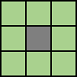Current pixel is shown in gray.

Three-dimensional connectivities

6

6-connected neighborhoodCurrent pixel is center of cube.

18

18-connected neighborhood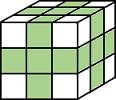Current pixel is center of cube.

26

26-connected neighborhood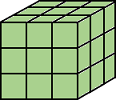Current pixel is center of cube.

Data Types: `single` | `double` | `int8` | `int16` | `int32` | `int64` | `uint8` | `uint16` | `uint32` | `uint64`

Maximum number of iterations performed by the algorithm. The algorithm can converge to a solution before reaching the maximum number of iterations.

Data Types: `single` | `double` | `int8` | `int16` | `int32` | `int64` | `uint8` | `uint16` | `uint32` | `uint64`

## Output Arguments

collapse all

Segmented image, returned as binary image of the same size as the label matrix `L`.

## Tips

• For `double` and `single` images, `grabcut` assumes the range of the image to be `[0 1]`. For `uint16`, `int16`, and `uint8` images, `grabcut` assumes the range to be the full range for the given data type.

• For grayscale images, the size of `L`, `foremask`, and `backmask` must match the size of the image `A`. For color and multi-channel images, `L`, `foremask`, and `backmask` must be 2-D arrays with the first two dimensions identical to the first two dimensions of the image `A`.

## Algorithms

• The algorithm treats all subregions fully or spatially outside the ROI mask as belonging to the background. To get an optimal segmentation, make sure the object to be segmented is fully contained within the ROI, surrounded by a small number of background pixels.

• Do not mark a subregion of the label matrix as belonging to both the foreground mask and the background mask. If a region of the label matrix contains pixels belonging to both the foreground mask and background mask, the algorithm effectively treats the region as unmarked.

• The algorithm assumes all subregions outside the region of interest belong to the background. Marking one of these subregions as belonging to foreground or background mask has no effect on the resulting segmentation.

 Rother, C., V. Kolmogorov, and A. Blake. "GrabCut - Interactive Foreground Extraction using Iterated Graph Cuts". ACM Transactions on Graphics (SIGGRAPH). Vol. 23, Number 3, 2004, pp. 309–314.

Download ebook